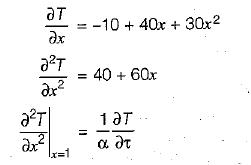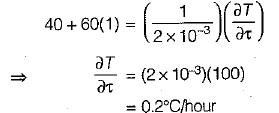Courses

# Test: Transient Heat Conduction - 2

## 8 Questions MCQ Test Topicwise Question Bank for Mechanical Engineering | Test: Transient Heat Conduction - 2

Description
This mock test of Test: Transient Heat Conduction - 2 for Chemical Engineering helps you for every Chemical Engineering entrance exam. This contains 8 Multiple Choice Questions for Chemical Engineering Test: Transient Heat Conduction - 2 (mcq) to study with solutions a complete question bank. The solved questions answers in this Test: Transient Heat Conduction - 2 quiz give you a good mix of easy questions and tough questions. Chemical Engineering students definitely take this Test: Transient Heat Conduction - 2 exercise for a better result in the exam. You can find other Test: Transient Heat Conduction - 2 extra questions, long questions & short questions for Chemical Engineering on EduRev as well by searching above.
QUESTION: 1

### The value of Biot number is very small (less then 0.1)

Solution:

Since Biot number is the ratio of conduction resistance to convection resistance and in lumped heat analysis convection resistance ≫ conduction resistance, it means that conduction resistance of solid is negligible as compared to convection resistance of fluid.

QUESTION: 2

### The time constant of a thermocouple is the time taken to attain

Solution:

Time constant of the thermocouple represents the time required for the thermocouple to record 63.2% of initial temperature difference.

QUESTION: 3

### Quenching is

Solution:
QUESTION: 4

In a lumped parameter model, the temperature variation with time is

Solution: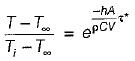Hence temperature variation will be exponential.

QUESTION: 5

One dimensional unsteady state heat transfer equation for a sphere with heat generation at the rate q can be written

Solution:
QUESTION: 6

Transient three-dimensional heat conduction is governed by ONE of the following differential equations (α - thermal diffusivity, k - thermal conductivity and ψ - volumetric rate of heat generation)

Solution:

Fourier equation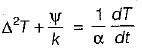QUESTION: 7

Steady two-dimensional heat conduction takes place in the body shown in the figure below. The normal temperature gradients over surface Pand Q can be considered to be uniform. The temperature gradient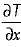at surface Q is equal to 10 K/m. Surface Pand Q are maintained at constant temperatures as shown in the figure, while the remaining part of the boundary is insulated. The body has a constant thermal conductivity of 0.1 W/mK. The value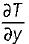and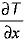at surface P are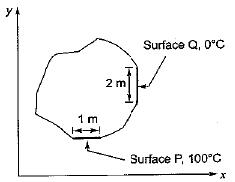Solution:
QUESTION: 8

A large concrete slab 1 m thick has one dimensional temperature distribution:
T = 4 - 10x + 20x2 + 10x3
where T is temperature and x is distance from one face towards other face of wail. If the slab material has thermal diffusivity of 2 x 10-3m2/hr, what is the rate of change of temperature at the other face of the wall?

Solution: